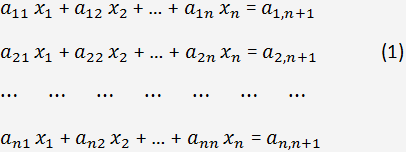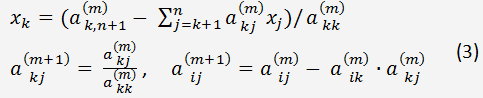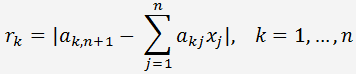# Solving systems of linear equations by Gaussian elimination

Solving systems of linear equations with determinants can be used for systems of two or three equations. For systems with more than three equations it is better to use the Gaussian elimination.

Gaussian Elimination is based on exclusion of unknowns. First we should use elementary row operations to reduce the matrix to row echelon form and then use the back substitution to solve each of the equations.

Consider the system of equations:where xi are the unknowns, i = 1, 2, ..., n; n < 200. If the number of equations is more than 200, it is necessary to use iterative methods.

ai,j are the elements of the augmented matrix of coefficients.

#### Gaussian elimination to reduce a system to triangular

Express x1 from the first equation
(2) x1 = (a1,n+1 - a1,2x2 - ... - a1nxn)/a11

If a1,1=0 and there is a non-zero element in the first column, we should swap rows. Then substitute (2) into all equations of the system (1), except the first equation. Thus, the unknown x 1 will be eliminated from all the equations of the system, except the first equation.

The elements of the augmented matrix can be calculated by the formulas:

a1j(1) = a1j/a11

aij(1) = aij - ai1a1j(1), i = 2,3,...,n; j = 1, 2, ..., n+1

After eliminating x1 from all the equations, all elements of the first column of the transformed matrix will be zero, except a11(1) = 1.

Repeat this procedure for x2 - express it from the second equation and eliminate it from the rest of equations, etc.

Doing this we will put the system into triangular form where all the elements below the main diagonal are zeros.

To eliminate xk.Next, we do a backward elimination to solve the linear system and find the unknowns xk from xn to x1. This procedure is called a backward elimination.

To minimize the error of division by the diagonal element in (3), it is recommended to rearrange the equations to put the element with the largest absolute value on the diagonal. This modification is called Gaussian elimination with partial pivoting.

To estimate the error of numerical solution of the system with discrepancies, the numerical solutions xk , k = 1, 2, ..., n must be substituted into the system. Then we should calculate the difference between the right and left parts of the equations.For small values of errors, r k will be zero.